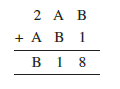# Q9. Find the values of the letters in each of the following and give reasons for the steps involved.The additiion of B and 1 is 8  is giving a number whose ones digit is 8. this means digit B is 7 .

B + 1 = 8 and no carry for next step.

next step :

Now, A + B = 1 => A + 7

which implies A = 4

A  + B = 11 and 1 is carry for next step

1 + 2 + A  = B

1 + 2 + 4 = 7

Hence A = 4 and B = 7 is correct answer.

## Related Chapters

### Preparation Products

##### JEE Main Rank Booster 2021

This course will help student to be better prepared and study in the right direction for JEE Main..

₹ 13999/- ₹ 9999/-
##### Rank Booster NEET 2021

This course will help student to be better prepared and study in the right direction for NEET..

₹ 13999/- ₹ 9999/-
##### Knockout JEE Main April 2021 (Easy Installments)

An exhaustive E-learning program for the complete preparation of JEE Main..

₹ 4999/-
##### Knockout NEET May 2021

An exhaustive E-learning program for the complete preparation of NEET..

₹ 22999/- ₹ 14999/-